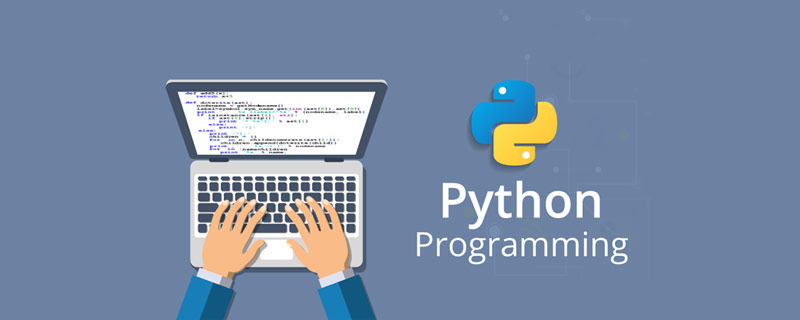Jquery中文网 www.jquerycn.cn
Jquery中文网 >  Python编程  >  python进阶  >  正文 python如何计算数的阶乘

# python如何计算数的阶乘

jquery中文网为您提供python如何计算数的阶乘等资源，欢迎您收藏本站，我们将为您提供最新的python如何计算数的阶乘资源

python计算数的阶乘的三种方法：1、使用“for i in range()”循环语句求阶乘；2、使用“reduce()”函数求阶乘；3、通过递归求阶乘。```a = 1
n = 5
for i in range(1,n 1):
a = a * i
print(a)```

`120`

```from functools import reduce
n = 5
print(reduce(lambda x,y:x*y,range(1,n 1)))```

`120`

```def factorial(n):
if n == 0 or n == 1:
return 1
else:
return (n*factorial(n-1))
a = factorial(5)
print(a)```

`120`

[]